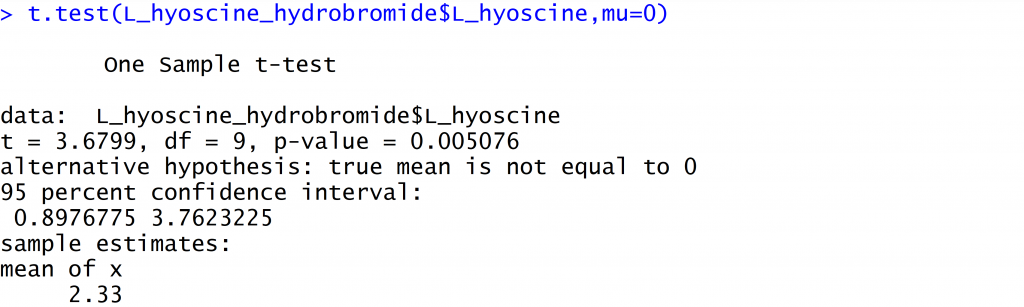# How to report a P-value

You will routinely find P-values in the output of statistical software.  How should they be reported?

### An example

If you've suffered from motion sickness, you might have taken a medication (such as "Kwells") based hyoscine hydrobromide.

In the early 20th century, the effect of hyoscine hydrobromide on sleep was investigated. In his original paper deriving the t-distribution, Student illustrated his methods using data from a study looking at the gain in hours of sleep after using various forms of hyoscine hydrobromide.  Here is a dotplot of the hours gained when the patients used a particular form - Laevo-hyoscine hydrobromide.  The measurement is the hours gained - the difference between using Laevo-hyoscine hydrobromide and not using it.

Here we consider carrying out a single sample t-test of the null hypothesis that the true mean hours gained was zero.

### Some output

Here are the results from various software pages.

### R### How you might see the results reported, none of which are recommended

• P = 0.0050706
• Too many decimal places!
• 0.005 in a table labelled "Sig."
• It's the P-value, not "Sig."
• P < 0.05
• That's only the ball park - the value in relation to an arbitrary threshold.
• Using the "star" system, and indicating that the result is less by 0.01 by using **.
• Again, that's only the ball park.
• Stating that the result is statistically significant.
• That's the implied ball park!  That only has implied numerical meaning.

### How to report P-values

• If you are reporting P-values in an academic paper or thesis, it's good practice to report the actual value to three decimal places.
• If the P-value is very small, common practice is to report it as P < 0.001.
• It's not sufficient to only report a P-value; relevant estimates and confidence intervals should also be provided.

For example, patients gained an average of 2.3 hours of sleep when using Laevo-hyoscine hydrobromide, with a 95% confidence interval of 0.9 to 3.8 hours. The P-value for a test of the null hypothesis that the true average gain was zero hours was P = 0.005.

### Student's paper

Student (1908) The probable error of the mean. Biometrika, 6, 1-25.